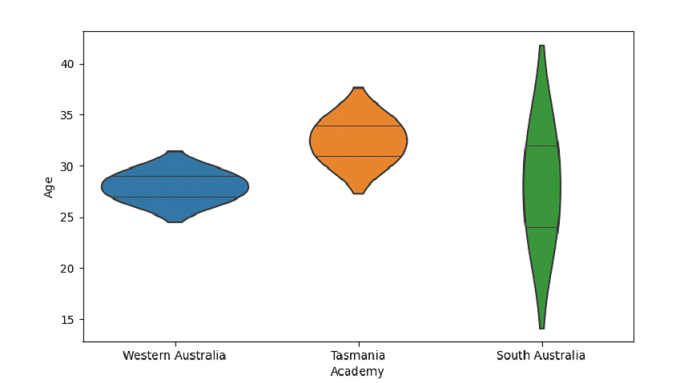# Python Pandas - Draw a violin plot, explicit order and show observation as a stick with Seaborn

PythonServer Side ProgrammingProgramming

Violin Plot in Seaborn is used to draw a combination of boxplot and kernel density estimate. The seaborn.violinplot() is used for this. Observations show as a stick using the inner parameter with value stick.

Let’s say the following is our dataset in the form of a CSV file − Cricketers.csv

At first, import the required libraries −

import seaborn as sb
import pandas as pd
import matplotlib.pyplot as plt

Load data from a CSV file into a Pandas DataFrame −

dataFrame = pd.read_csv("C:\\Users\\amit_\\Desktop\\Cricketers.csv")


Plotting violin plot with Academy and Age. Control order by passing an explicit order i.e. ordering on the basis of "Academy". Observations show as a stick using the inner parameter with value stick −

sb.violinplot(x = 'Academy', y = "Age", order=["Western Australia", "Tasmania","South Australia"],data = dataFrame, inner="stick")

## Example

Following is the code −

import seaborn as sb
import pandas as pd
import matplotlib.pyplot as plt

# Load data from a CSV file into a Pandas DataFrame:

# plotting violin plot with Academy and Age
# Control order by passing an explicit order i.e. ordering on the basis of "Academy"
# observations show as a stick using the inner parameter with value stick
sb.violinplot(x = 'Academy', y = "Age", order=["Western Australia", "Tasmania","South Australia"],data = dataFrame, inner="stick")

# display
plt.show()

## Output

This will produce the following output −